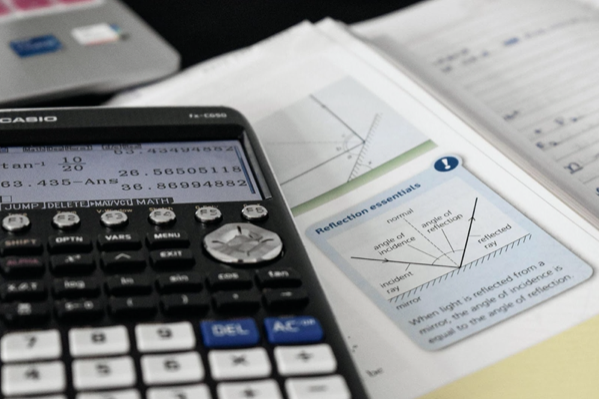In this article, we consider what knowledge of trigonometry will be helpful for studying physics in college and give some useful tips and resources for learning the subject.

## Physics College Preparation: The Easy Way to Learn Trigonometry

Are you going to go to college and study physics? Then you will definitely need knowledge of trigonometry. The more you know about it and algebra, the easier it will be for you to study physics in the future because the two fields are closely related. What kind of knowledge do you need first, and how to study trigonometry, we will discuss this in the article.

A physics problem is often a complex in which places and things interact. To solve this, you will need to draw a diagram. After a while, you will notice that in many diagrams for movement in two dimensions, right triangles show up. And, of course, you will need trigonometry knowledge to solve such problems. And not only here, but physics also requires it in numerous instances. So, you can't do without studying this discipline.

But what to do when your knowledge is not at a high level? Of course, do extra. There are many opportunities for this on the Internet: you can find study materials and assignments.

So if you have issues with finding solve solutions for any trigonometry difficulty, you can always turn to math sites or forums for help. There you can find information about how to understand the principle of the discipline and how to solve trigonometry problems. Furthermore, you can ask your questions to other users to help you resolve them or give some hints on how to do it.

But besides trigonometry, what other skills and knowledge do you need for physics in college? Let's look into it.## What do you need to get into a physics college?

Each college has its own recommendations on subjects high school students should take. Therefore, if you have already chosen an educational institution, follow this list. And don't be fooled by the fact that this is just a recommendation. Colleges want to see this list of all subjects, after all, your academic success is one of the main criteria for admission.

## What courses do colleges in physics usually require?

A-level subjects that are needed or essential for physics:

• maths;
• physics.

Useful for physics A-level subjects are:

• further maths;
• chemistry.

Also, it will be a good idea to take an advanced placement in:

• Calculus;
• Physics;
• Chemistry;
• Computer Science.

If you had a Maths Mechanics module in your course, it would also be a plus. But first of all, you should focus on the college you have chosen and try to meet their requirements for 100%, even if they are only a recommendation.

## How to learn to solve trigonometry problems and study the discipline?

Of course, there is no one-size-fits-all method, you have to find one that works for you. Here are some examples and tips on how to learn trigonometry for newbies and for advanced students.

## General rules

• After each lesson, walk through the material at home again, ensuring you understand everything.
• Before the lesson, read the textbook with a new topic, and follow the examples. You don't need to understand them fully, just get an idea of what you are going to learn.

## How to study trigonometry formulas step by step

1. Memorize all the basics of trigonometric angles.
2. Learn what a right triangle is.
3. Pythagoras' theorem: you need to know it by heart.
4. Next, pay attention to the rule of sine and cosine.
5. Remember all the essential accuracy of trigonometry.
6. Study the trigonometry table thoroughly. It contains the values of trigonometric relations of standard angles.

It will be easier for you to learn trig if you know all the formulas and practice a lot. You can go back to last year's tasks, start with the simple ones and move on to the more complex ones. This will help you improve your speed.

## Resources for learning trigonometry and practice in solving

• Quora. An excellent way to get an answer is if you have a specific theoretical question. The site has many graduates from various colleges, including those who have studied trigonometry in physics.
• Khan Academy. It has different tasks, you can focus on a specific topic, and points are given for passing the topics.
• Symbolab. A site where you can solve problems and test yourself right away. You can practice the graphics as well.
• Bitesize from the BBC contains lessons, formulas, and tests on trigonometry.

## Conclusion

You have chosen not an easy path if you decide to study physics. But if solving complex problems in mathematics and physics is something you like and would like to develop in it, then this is the right decision. And learning the rules of trigonometry is the first step to a dream. Good luck!